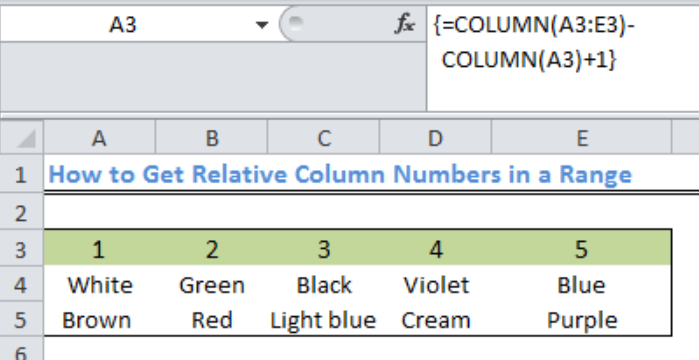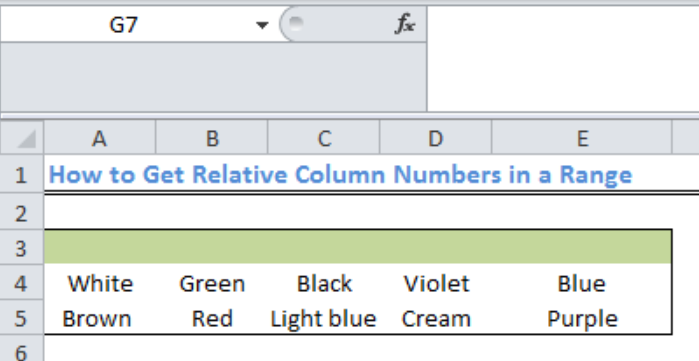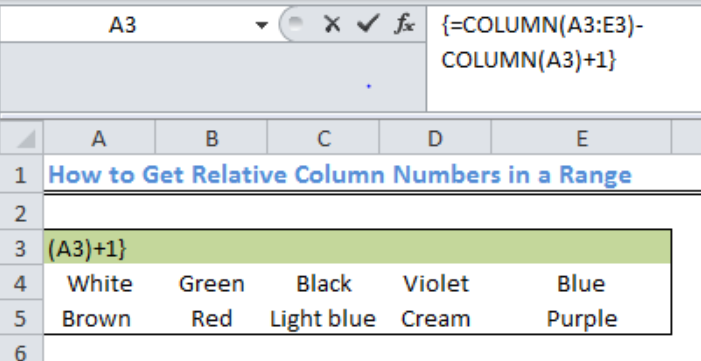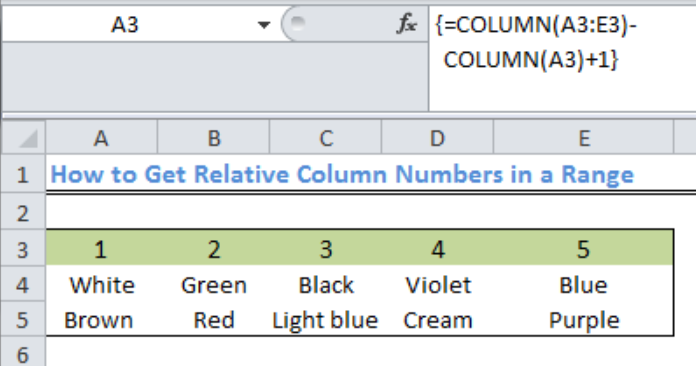Get instant live expert help with Excel or Google Sheets“My Excelchat expert helped me in less than 20 minutes, saving me what would have been 5 hours of work!”

#### Post your problem and you’ll get expert help in seconds.

Your message must be at least 40 characters
Our professional experts are available now. Your privacy is guaranteed.

# How to Get Relative Column Numbers in a Range

We can get a complete set of relative column numbers in a range with an array formula that is based on the COLUMN function. The steps below will walk through the process.Figure 1: Result of Relative Column Numbers in a Range:

## General Formula

`{=COLUMN(range)-COLUMN(firstcell)+1}`

## Formula

`=COLUMN(A3:E3)-COLUMN(A3)+1`

## Setting up the Data

We will determine the relative column number for range A3:E3 based on the content of figure 2Figure 2: Setting up the Data

## Get Relative Column Numbers in a Range

• We will highlight the range from Cell A3 to E3
• We will input the formula below inside the range

`{=COLUMN(A3:E3)-COLUMN(A3)+1}`Figure 3: How to Get Relative Column Numbers in a Range

• Because this is an array formula, we will press CTRL+SHIFT+ENTER to get the resultFigure 4: Result of Relative Column Numbers in a Range

## Explanation

In this formula, the first Column function creates an array of 5 numbers like this: {2,3,4,5,6}. The second Column function creates an array that has only one item: {2}. This is subtracted from the first array and results in {0,1,2,3,4}. 1 is added to the result to produce: {1,2,3,4,5}.

## Instant Connection to an Expert through our Excelchat Service

Most of the time, the problem you will need to solve will be more complex than a simple application of a formula or function. If you want to save hours of research and frustration, try our live Excelchat service! Our Excel Experts are available 24/7 to answer any Excel question you may have. We guarantee a connection within 30 seconds and a customized solution within 20 minutes.

### Did this post not answer your question? Get a solution from connecting with the expert.Another blog reader asked this question today on Excelchat:
Solution examplesHello, I need help regarding indirect formula of excel. 1. What is the purpose of using this formula? 2. If we use this formula with sum formula, then why we are using this with sum formula, we can also perform addition from different sheets without indirect formula ?
Solved by O. D. in 21 minsneed help how to add a percentage to a column and subtract
Solved by B. U. in 43 minsHello, I need help on sorting a rather large list. It is data with people's names and things they purchased. COLUMN A: Items Purchased COLUMN B: FIRST NAME COLUMN C: LAST NAME For every item purchased, is another row even if it's the same person. So: Doll Abbi Sumandal Toy Abbi Sumandal I'm trying to find a way for Excel to recognize duplicates in columns B&C (Abbi Sumandal) and take the unique values and set them in the same row. Final: Abbi Sumandal Doll Toy Is there a formula or function that can do this?!
Solved by V. H. in 26 minsHOW CAN I MAKE A TALE FOR ONLY 6 COLUMN?
Solved by Z. A. in 40 minsi NEED TO SUMM UP TO ONE THOUSAND ENTRIES IN A COLUMN
Solved by T. W. in 18 mins## Subscribe to Excelchat.coAnother blog reader asked this question today on Excelchat: